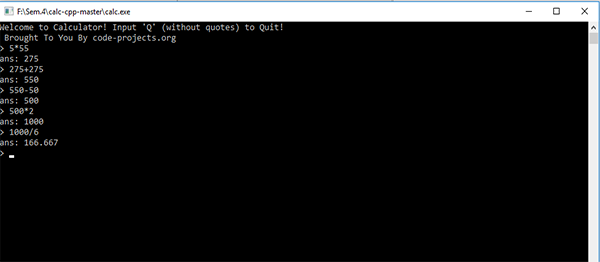## CALCULATOR IN C++ WITH SOURCE CODE

This project is based on a concept to generate the total sum, subtractions, divisions and multiplication. User has to input numbers with mathematical signs (“+,-,*,/”), the system will display the output. [ user has to input : 5*5, then the system will d...

Type : Project

File Size : 451.9 KB# Similar Projects and Reports

#### CALCULATOR IN C++ WITH SOURCE CODE

This project is based on a concept to generate the total sum, subtractions, divisions and multiplication. User has to input numbers with mathematical signs (“+,-,*,/”), the system will display the output. [ user has to input : 5*5, then the system will d...

#### Calculator App In C# With Source Code

This Calculator App in C# is based on the concept of normal mathematical operations on the calculator. The project contains the numbers, operators, and signs like in a normal calculator. Here, the user cannot enter the number themselves, they can just cli...

#### Simple Calculator In C# With Source Code

Simple Calculator in C# is based on the concept of normal mathematical operations on the calculator. The project contains the numbers, operators, and signs like in a normal calculator. So, the user cannot enter the number themselves, they can just click o...

#### Advance Calculator In C++ With Source Code

The Advance Calculator is based on the concept of different mathematical calculations. This whole system is in the C++ language. Here, the user can perform various calculations like arithmetic, trigonometric, exponential, and logarithmic. Also, it is a ve...

#### CALCULATOR IN PYTHON WITH SOURCE CODE

This Calculator project In Python is a simple project developed using Python. The project contains the numbers, operators, and sign like in normal calculator. So, the user can enter any number they want in the calculations. Hence, the user can use a simpl...

#### Voice Calculator App In C# With Source Code

Voice Calculator App in C# is based on the concept of voice-over mathematical operations on the calculator. The project contains the numbers, operators, and signs like in a normal calculator. Here, the user can also enter the number themselves for the cal...